## Introduction

The USGS NEIC provides Finite Fault Models for larger earthquakes. An example of this is at href="http://earthquake.usgs.gov/earthquakes/eqinthenews/2012/usc000cfsd/finite_fault.php">http://earthquake.usgs.gov/earthquakes/eqinthenews/2012/usc000cfsd/finite_fault.php.

This tutorial shows how to use the Computer Programs in Seismology codes to compute  teleseismic vertical component P-wave synthetics, regional vertical north and east (ZNE) synthetics and finally  static deformation from the published finite-fault solution.

The finite fault model is that of the Costa Rica earthquake of 2012/09/05 14:42:00, which is the subjet of the first tutorial.

This tutorial provides a unified organization to organize and compute teleseismic P-waves, regional waveforms an regional deformation

## Requirements

To run the simulations, you will need the following:
• Unpack the FINITE_FAULT.tgz
• gunzip -c FINITE_FAULT.tgz | tar xf -       which will give the following directory structure
FINITE_FAULT/
|------index.html
|-----------------Models/                          [Velocity models]
|                            |----tak135sph.mod
|                            |----CUS.mod
|                            |----WUS.mod
|
|----FiniteFaultModels/
|                             |----HayesCostaRica.mod    [Rupture model for Costa Rica earthquake 2012/09/05]
|
|----------FINITE.TEL/
|                             |-----index.html
|                             |-----DOIT.TEL
|----------FINITE.REG/
|                             |-----index.html
|                             |-----DOIT.REG
|------FINITE.STATIC/
|                             |-----index.html
|                             |-----DOIT.STATIC
• A list of stations for which synthetics are to be made
• the finite fault model given by a link at the bottom of the USGS Finite Fault solution page
• NOTE: The DOIT.TEL and DOIT.REG scripts use the gsac map comand to produce map.sh which can be used for a GMT3/GMT4 installation. If you have GMT5 installed, then edit these scripts to change the lines
`map ksntm on global on ray onsh map.sh# if GMT5 is installed comment the previous line and then # uncomment the next two#map5 ksntm on global on ray on#sh map5.sh`
`#map ksntm on global on ray on#sh map.sh# if GMT5 is installed comment the previous line and then # uncomment the next twomap5 ksntm on global on ray onsh map5.sh`

## Simulations

I assume that you are in the top level directory FINITE.

### Teleseismic

`    cd FINITE.TEL    DOIT.TEL`
This simulation computes teleseismic P-wave signals using the tak135sph.mod model and the HayesCostaRica.mod rupture model.  The DOIT.TEL creates the subdirectories WORK, STACK and FINAL in this directory. The stacked teleseismic synthetics are Sac files in the subdirectory FINAL. This example creates the following files:

`ADK.Z	ASCN.Z	CMB.Z	EFI.Z	GRFO.Z	JOHN.Z	KEV.Z	KONO.Z	NV31.Z	PD31.Z	POHA.Z	SHEL.Z	TRQA.ZAFI.Z	BFO.Z	CMLA.Z	ESK.Z	HOPE.Z	KBS.Z	KIP.Z	MACI.Z	PAB.Z	PFO.Z	RSSD.Z	SSPA.Z	TUC.ZANMO.Z	BORG.Z	COR.Z	FFC.Z	HRV.Z	KDAK.Z	KNTN.Z	MIDW.Z	PASC.Z	PMSA.Z	SFJD.Z	TRIS.Z`
The DOIT.TEL also creates the graphics files map.png and finite_tel.png which are shown in the next figure: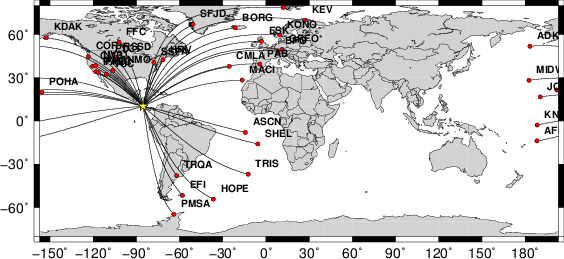Location of epicenter and paths to stations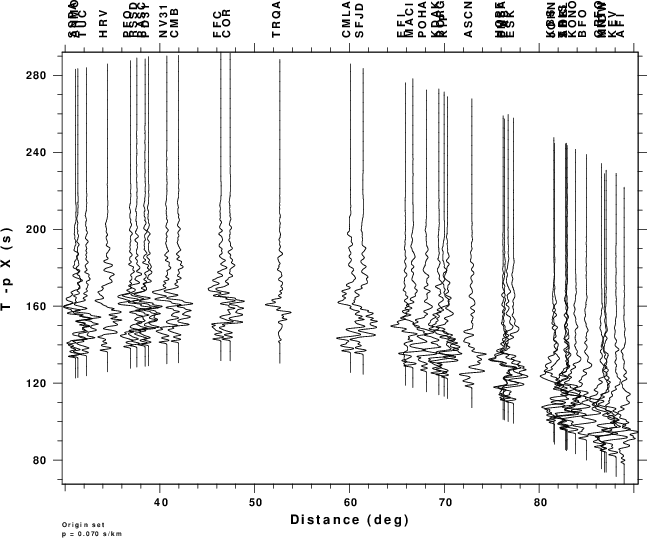Teleseismic P-wave simulations of ground velocity in m/s from the finite fault

### Regional

`    cd FINITE.REG    DOIT.REG`
This simulation computes regional  signals using the WUS.mod model and the HayesCostaRica.mod rupture model.  Because wavenumber integration is used, the computations will be lengthy. So the control file, dfile, was created as follows for each subfault to each station

`cat > dfile << EOF\${DIST} 1.0 512 -20 8.5EOF`
The 1.0 indicates a sample rate of 1.0 sec, the 512 indicates the number of points in the time series, and the first time sample is given by  t = -20 + DIST/8.5.  The computational time is such that if I had used 0.5 sec and 1024 points to include higher frequencies, then the time woudl increase by a factor of 4. I thought that my chose here was appropriate since the finite fault model was constructed using long period data.

The DOIT.REG creates the subdirectories WORK, STACK and FINAL in this directory. The stacked teleseismic synthetics are Sac files in the subdirectory FINAL. This example creates the following files:

`BCIP.E	BCIP.N	BCIP.Z	JTS.E	JTS.N	JTS.Z`
The DOIT.REG also creates the graphics files map.png and finite_tel.png which are shown in the next figure: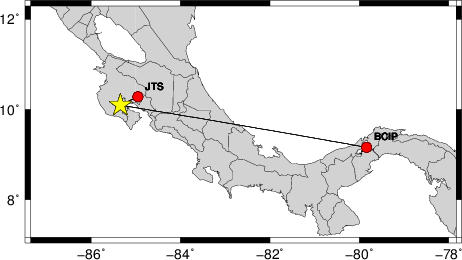Location of epicenter and paths to stations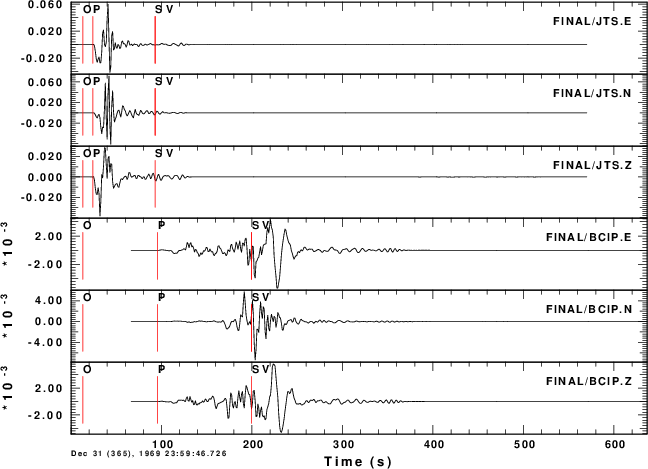Regional simulations of ground velocity in m/sfrom the finite fault

### Static deformation

`    cd FINITE.STATIC    DOIT.STATIC`
This simulation computes static deformation for the WUS.mod model and the HayesCostaRica.mod rupture model. Since hstat96 computes the solution for a multilayered halfspace through numerical integration, this example runs a long time. On the other hand if hsanal96 is used to compute the halfspace solution, the computations are then very, very fast.

Two sets of GMT plots are created. The first is a direct contour of the deformation values, and the second is a contour plot after applying the surface command of GMT.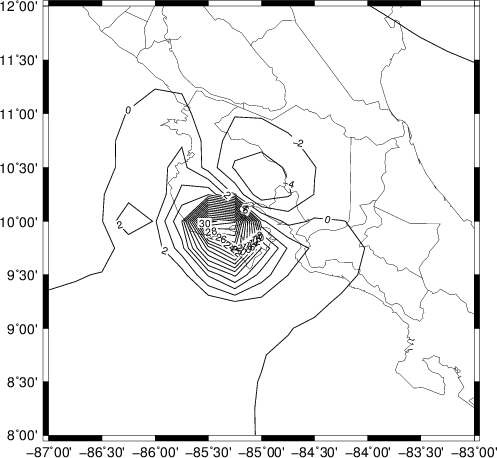Z (units cm) using GMT xyz2grd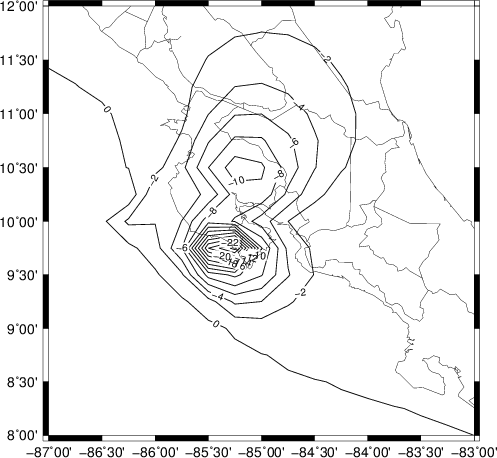N (units cm) using GMT xyz2grd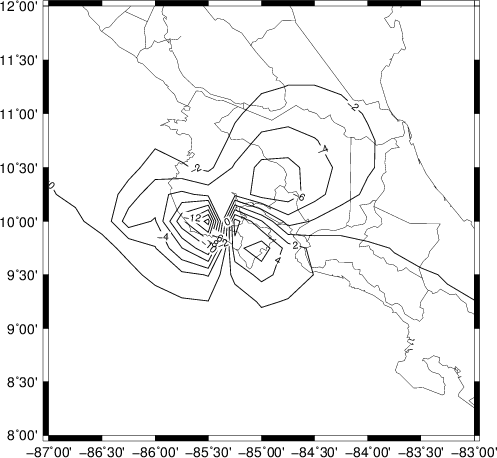E (units cm) using GMT xyz2grd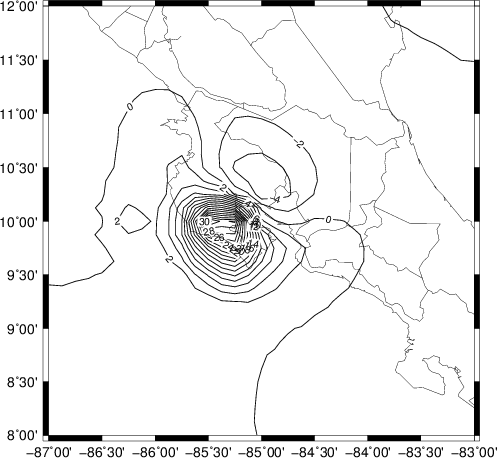Z (units cm) using GMT surface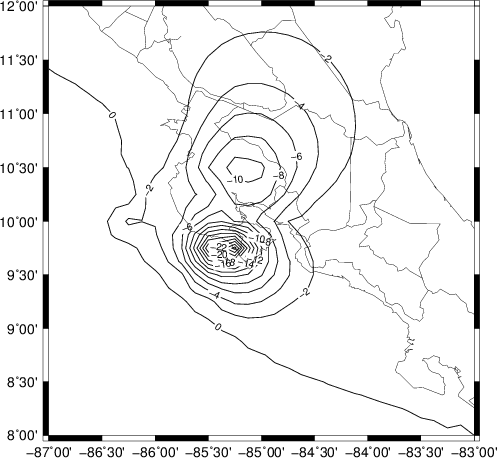N (units cm) using GMT surface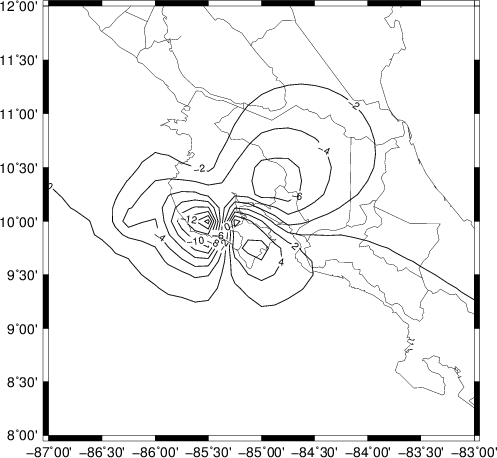E (units cm) using GMT surface